This is an old revision of the document!

## Lab 05. Connecting Analog Sensors to the board and adding charts

At the end of this project you will know how to get data from different sensors you can place around your home and display the gathered data on graphs.

In case you want to use a Raspberry Pi, please note that you will need to use an Arduino as well. No analog pin dependent piece can be used with your Raspberry Pi only. You will need the help of an Arduino board.

Let's begin with the temperature sensor.

### Build a virtual thermometer

#### What you need

• One temperature sensor;
• Three jumper wires;
• One Edison connected to Wyliodrin.

#### The setup

In this figure you can see a 3-leg temperature sensor. There is an alternative to this one, the thermistor. The latter has two legs and it should be connected as a voltage divider, which is explained in the electronics chapter.

In this case, the sensor needs to be connected to the GND pin, the VCC and an analog pin. This sensor consists of a thermistor and several other electronic pieces. The leg situated in the middle outputs a different voltage depending on the temperature.

#### The Code

In order to get the values from the the temperature sensor you will just have to use the usual Analog read blocks and read the analog pin. The values that you obtain are in a range of 0 to 1023. However, this doesn't look at all like the values you would expect from a thermometer.

To transform these values into Celsius degrees, you are going to use this formula.
temperature= (value*5/1024-0.500)*100
How does it look in Streams programming language? You need no more than 3 nodes.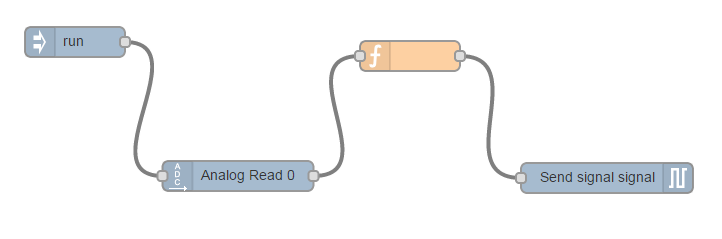The first mandatory one, which is run. The next one will read the value that comes from the analog pin, in this case A0. Now the payload of the message sent will be this very value, between 0 and 1023. Now, you have to convert it to Celsius degrees.

To get from this value to Celsius degrees you have to follow the formula above. The resulting value is stored in the temperature variable.

To write the formula you can use either a visual node or a formula node. Choose the latter and the code inside should look like the one in the next figure.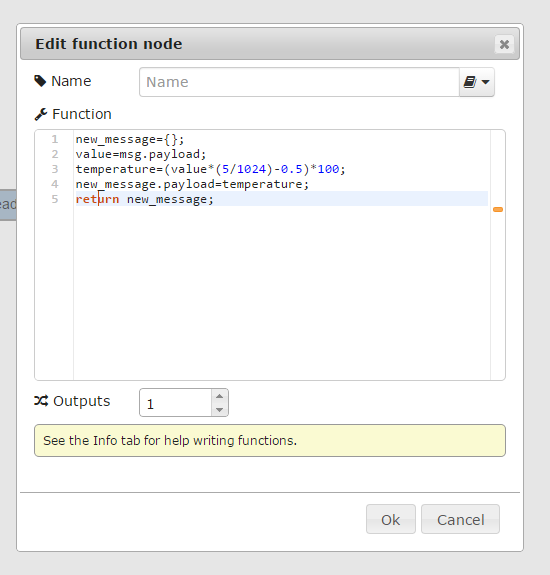As promised, you will now display the temperature on a thermometer using the send signal node.

In dashboard choose the thermometer and assign it a signal with the same name as use the nodes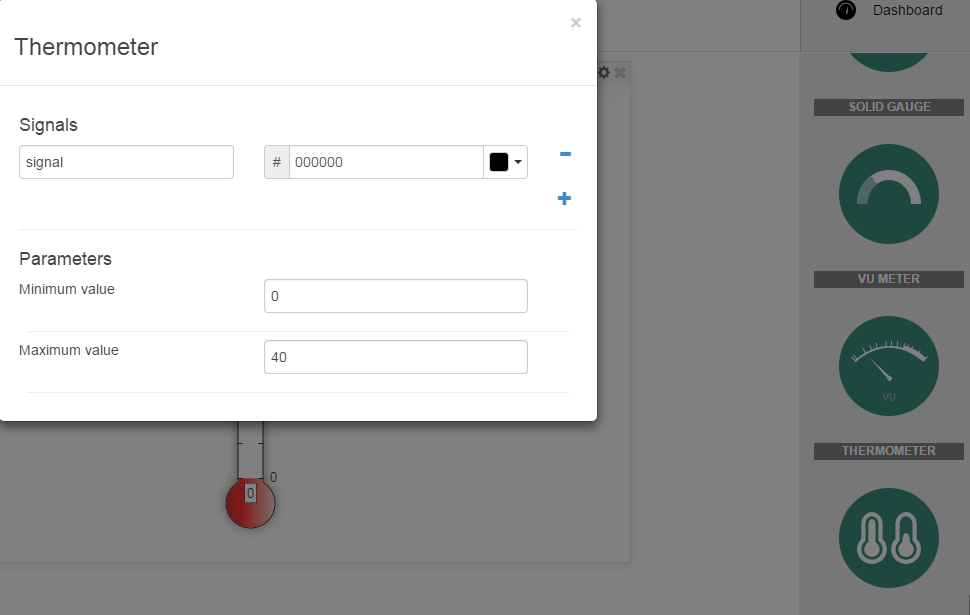The send signal node stores the value it receives from the previous block and sends it further to the dashboard, where a graph with the exact same name as the node's signal is searched. Once found, the connection is established. The node will receive the signal the data, store it and send it to the graph via a signal.

#### Use a thermistor

As mentioned at the beginning, the same project can be easily built with a thermistor.

Note that there is a difference between the two sensors, consisting of the fact that the temperature sensor previously used has a linear resistance-temperature characteristic, while the other one usually has the output voltage as a logarithmic or exponential function.

The wiring is quite simple, just follow the schematics of a voltage divider.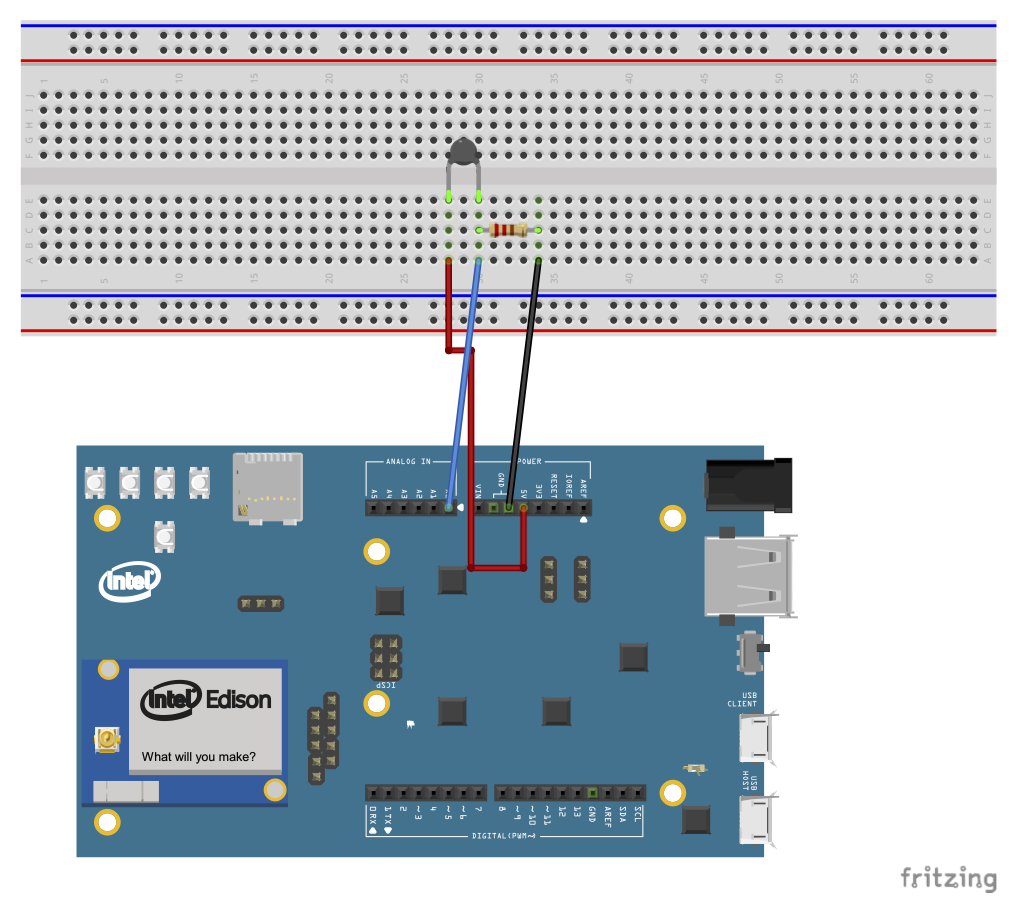For the code you will need to know that this time the formulas you use are different to convert the values into Centigrade.
R_t = 5/V_m * 10000
Ratio = 1/B* ln(R_t/10000)
temperature = 1/(1/298 + Ratio)
B = 4050, is one of the constants that comes with the sensor's specifications.

Vm is the voltage that you get in your analog input while Rt is the resistance of the thermistor. By having this value, you just have to apply the following formulas and you will obtain the temperature in Celsius degrees. You only have to calculate what the formulas above will give and put it in a variable. Send the value of this variable to the thermometer in the Dashboard. The only difference will be in the function that you use to do the conversion.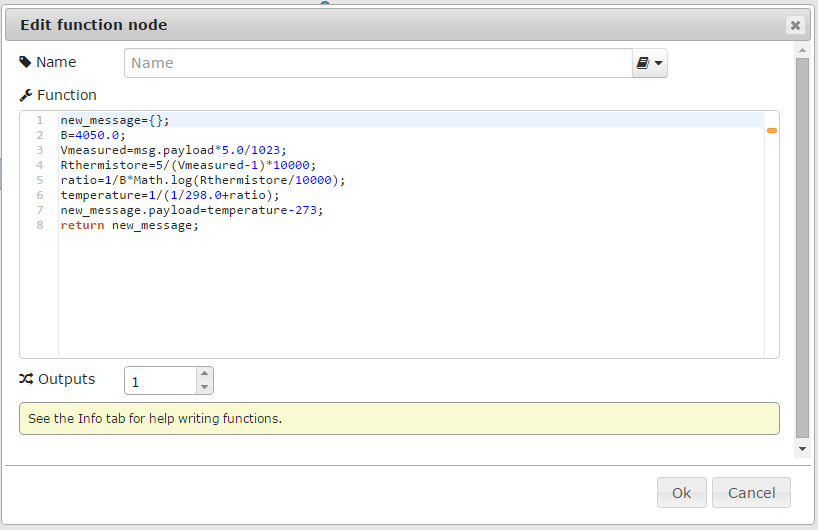In Visual Programming, the same function would look like this: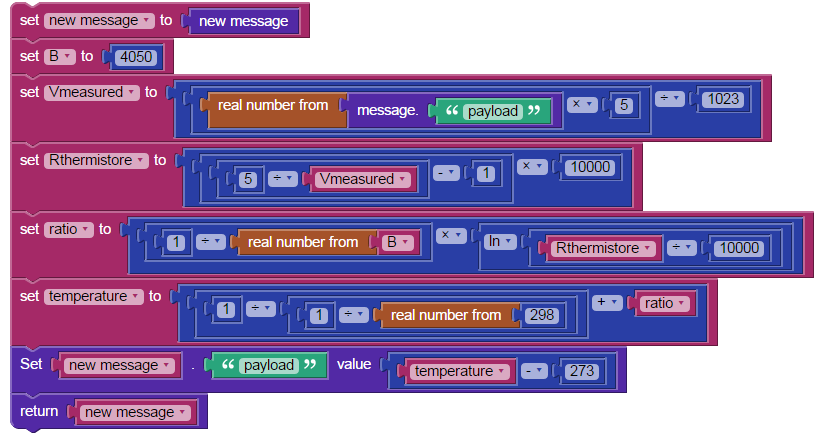### See how the light changes in a room

You that now have a thermometer can you find something extra to make it more interesting? Try a light sensor.

• One photocell;
• One 220 $\Omega$ resistor;
• Three jumper wires.

#### The Setup

The photocell works just like a voltage divider, as well (check the Introduction to Electronics chapter for more details). You need to connect it to the Gnd and Vcc and from between the photocell and the resistor to the analog pin of the board. This light sensor has a resistance that varies with the light, the more light there is, the lower the resistance of the photocell. Depending on how you place the sensor you can obtain a pull up or pull down voltage divider.

#### The Code

The new nodes are much simpler. You just add a block to read the analog pin that you use and send it to the chart.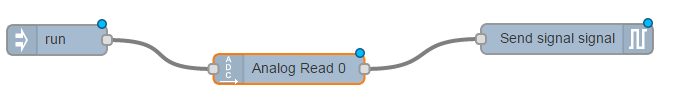### Exercises

1. Ordered List ItemConnect a photoresistor. Send the values to a chart. Set a maximum value. In case the sensor detects a value higher that the maximum, an LED will turn on for a few seconds.
2. * Do the same, but set the limit value from a slider, in the dashboard. This slider gives values between 0 and a maximum of your choice.
3. For this exercise you will have to make a chart using the values of a photoresistor as well, only that this time, the team next to you will have the sensor and they will send a signal with its values. You will receive this values and send them to the dashboard. In short, make a line chart using the values of a photoresistor connected to the neighbour's board.Hint In order to get a message from another board, you will need its Board ID. To find it, go to the main IOT page and in the boards settings, choose BoardID.
4. - Use a thermistor. You need to find online the value B and it's resistance at 25 degrees. Put its values in degrees in a thermometer chart.
Hint You will wire it as a voltage divider with a similar resistance.